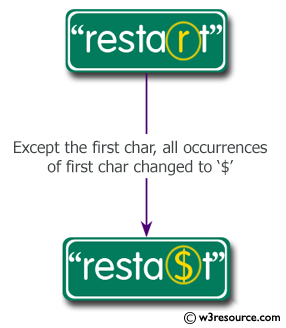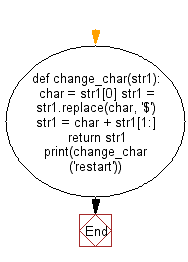﻿ Python: Get a string from a given string where all occurrences of its first char have been changed to '\$', except the first char itself - w3resource# Python: Get a string from a given string where all occurrences of its first char have been changed to '\$', except the first char itself

## Python String: Exercise-4 with Solution

Write a Python program to get a string from a given string where all occurrences of its first char have been changed to '\$', except the first char itself.Sample Solution:-

Python Code:

``````def change_char(str1):
char = str1
str1 = str1.replace(char, '\$')
str1 = char + str1[1:]

return str1

print(change_char('restart'))
```
```

Sample Output:

```resta\$t
```

Flowchart:## Visualize Python code execution:

The following tool visualize what the computer is doing step-by-step as it executes the said program:

Python Code Editor:

Have another way to solve this solution? Contribute your code (and comments) through Disqus.

What is the difficulty level of this exercise?

Test your Programming skills with w3resource's quiz.

﻿

## Python: Tips of the Day

What is the difference between Python's list methods append and extend?

append: Appends object at the end.

```x = [1, 2, 3]
x.append([4, 5])
print (x)
```

Output:

```[1, 2, 3, [4, 5]]
```

extend: Extends list by appending elements from the iterable.

```x = [1, 2, 3]
x.extend([4, 5])
print (x)
```

Output:

```[1, 2, 3, 4, 5]
```

Ref: https://bit.ly/2AZ6ZFq